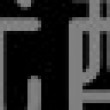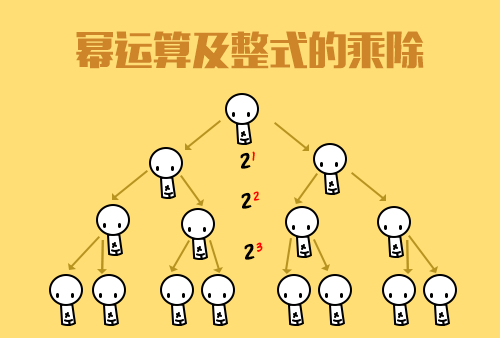| | |

||
APP端下载AndroidiPhone
￥249

|||

|
1537人点赞
752729人已学习
|

3天无理由退款

3天无理由退款：退款将以超级币形式退至您的超级课堂学习账户，便于您重新选购其他课程。恶意退款将被冻结账号。

• 1、会改变的量叫变量，数值固定不变的量就是常量
2、 函数是两个变量之间的的一种关系，自变量改变，因变量跟着发生改变
3、 一般地，在某一变化过程中有两个变量$x$和$y$，如果对于$x$的每一个值，$y$都有唯一确定的值与它对应，那么把$x$称作自变量，$y$称作因变量，$y$是$x$的函数
4、 "唯一”是说一个自变量只能对应一个因变量
• 1、解析法表示函数，就是把两个变量的函数关系用一个等式来表示，这个等式称作函数的解析式
2、 解析式中的自变量往往有一个取值范围，在求取值范围时要注意两方面的因素：解析式要有意义，同时还要符合实际意义
3、 初中阶段对于解析式的三种限定：分母不为零、二次根号下要大于等于零、指数为零则底数不为零。
4、 解析式是我们通向函数世界的最简洁的一座桥
• 1、函数有三种表示法—解析法，列表法和图象法，它们各有千秋，也各有缺憾
2、 列表法直观明了，但有很明显的缺陷，这就是表格的有限性
3、 所谓图象法，就是用图象表示两个变量之间的对应关系
4、 函数图象连续与否取决于自变量的取值特征
5、 图象法的优点，就是形象直观的表示函数的变化趋势
6、 图象法在表示函数变化趋势这方面最给力，但它在读数方面有极大的缺陷，由于误差，准确的值就无法知晓了
• 1、正比例函数的解析式：$y=kx$，$k$是常数，且$k\neq 0$。一个函数是正比例函数要满足三点：(1)、$k$是常数且不为零；(2)、$x$必须是一次；(3)、常数项是$0$
2、 正比例函数的定义域：全体实数。但很多题目中则要考虑实际情况，$x$一般是有具体限制的
3、 常见重要技巧：待定系数法求函数解析式
• 1、函数图象的画法，列表，描点，连线，就这三步
2、 正比例函数的图像特点——一条穿过原点的直线
3、 研究$k$对图像的影响。$k$的正负决定了倾斜方向，正数时，$x$和$y$的变化趋势一致，是增函数，图像向右倾斜，手心向上斜劈的方向。负数时，$x$和$y$的变化趋势相反，是减函数，图像向左倾斜，手背向上斜劈的方向
4、 直线的倾斜程度，要看$k$的绝对值。绝对值越大，直线越陡峭
• 1、在原点外确定一点，就可以画出正比例函数的图像，这个点一般是$\left ( 1,k \right )$
2、 通过原点外一点的待定系数就可以求出$k$
3、 $k$对于正比例函数的重要性，它确定了直线的旋转角度，正比例函数的直线就像螺旋桨一样，绕着原点旋转，靠$k$确定角度
• 1、一次函数的解析式：$y=kx+b$（$k$、$b$是常数，且$k\neq 0$），$k$叫斜率，$b$叫截距。它满足的两点：(1)，$k$是常数且$k$不为零,(2)，自变量$x$的指数是$1$
2、 一次函数与正比例函数的关系：一次函数包含正比例函数，正比例函数其实就是一种特殊的一次函数，常数项$b=0$的一次函数
3、 和正比例函数一样，一次函数的定义域也是全体实数，但实际问题要对定义域进行限定
4、 一次函数解析式的求法，还是待定系数法。为了解出$k$、$b$两个未知数，需要知道两组$x$、$y$的值，列方程组
• 1、一次函数的图像是一条直线，作图时把握两个特殊点就可以：$\left ( -\frac{k}{b},0 \right )$和$\left ( 0,b \right )$，分别是和$x$轴、$y$轴的交点
2、 一次函数中斜率$k$、截距$b$对图像的影响
3、 $k$决定直线的倾斜角度
4、 $b$决定直线与$y$轴的交点位置
5、 据$k$、$b$的正负就可以确定一次函数图象的大致位置,反过来也能根据图像推断$k$，$b$的正负
• 1、点的坐标满足某个函数的解析式，点就在这个函数的图象上
2、 把某点的坐标代入函数解析式，看等式是否成立，就能验证它在不在函数的图象上
3、 函数图象上任意一点的坐标一定满足解析式，所以利用解析式，可以设出函数图象上某一点的坐标
4、 求直线的交点，$y=k_{1}x+b_{1}$和$y=k_{2}x+b_{2}$，本质就是解方程组，解得的$x$和$y$分别是交点的横坐标和纵坐标
• 1、一条直线与两条坐标轴围成的三角形：令$x$和$y$分别为$0$，求出$B$的纵坐标和$A$的横坐标，然后取绝对值，乘积除以$2$就是面积
2、 两条直线和一条坐标轴围成的三角形：先求出两条直线交点的坐标，交点到相应坐标轴的距离就是高。然后分别求出两条直线与相应坐标轴的交点坐标，差的绝对值就是底长，底乘高除以$2$就是面积
• 1、平行的一次函数图像，他们的解析式特点。$l_{1}$：$y=k_{1}x+b_{1}$和$l_{2}$：$y=k_{2}x+b_{2}$；$k_{1}=k_{2}$且$b_{1}\neq b_{2}$
2、 平行的直线斜率相同，截距不同；反过来，斜率相同，截距不同的解析式，图像势必平行
3、 函数的平移规律：左加右减、上加下减。上加下减把$b$加上或减去移动的$m$个单位
4、 左加右减是把$x$整体换成$(x+m)$或$(x-m)$
• 1、垂直直线的解析式特点：当两直线$y=k_{1}x+b_{1}$和$y=k_{2}x+b_{2}$垂直时,斜率互为负倒数$k_{1} \cdot k_{2}=-1$
2、 反过来就是如何判定两直线是否垂直，只要$k_{1}\cdot k_{2}=1$，两直线就垂直

• 110000
• 2小头爸爸
• 3超级学员603254
• 4李晨曦
• 5超级学员743163
• 6超级学员805818
• 7哈哈哈哈
• 8Yoyo
• 9超级学员856383
• 10超级学员884340767546人在学
￥ 330 ￥ 1851306150人在学
￥ 210 ￥ 8756964人在学
￥ 330 ￥ 220755534人在学
￥ 270 ￥ 175

• 0
• 点击分享有好礼
•app端下载关注微信号